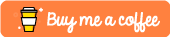# violinplotter

## Plotting and Comparing Means with Violin Plots

R package for generating violin plots with optional mean comparison with nonparametric Mann-Whitney test (default) or parametric Tukey’s honest significant difference as well as simple linear regression. This package aims to be a simple and quick visualisation tool for comparing means and assessing trends of categorical variables.

## Usage

``````violinplotter(formula, data=NULL, TITLE="", XLAB="", YLAB="", VIOLIN_COLOURS=c("#e0f3db", "#ccebc5", "#a8ddb5", "#7bccc4", "#4eb3d3", "#2b8cbe"), PLOT_BARS=TRUE, ERROR_BAR_COLOURS=c("#636363", "#1c9099", "#de2d26"), SHOW_SAMPLE_SIZE=FALSE, SHOW_MEANS=TRUE, CATEGORICAL=TRUE, LOGX=FALSE, LOGX_BASE=10, MANN_WHITNEY=TRUE, HSD=FALSE, ALPHA=0.05, REGRESS=FALSE)

## Installation

``install.packages("violinplotter")``

``````### install the "remotes" package to install R packages from github repositories
install.packages("remotes")
### install "violinplotter" (this command also performs package update)
remotes::install_github("jeffersonfparil/violinplotter")``````

## Examples

Simulated dataset:

``````library(violinplotter)
x1 = rep(rep(rep(c(1:5), each=5), times=5), times=5)
x2 = rep(rep(letters[6:10], each=5*5), times=5)
x3 = rep(letters[11:15], each=5*5*5)
y = rep(1:5, each=5*5*5) + rnorm(rep(1:5, each=5), length(x1)) ### x3 is the variable affecting y (see each=5*5*5)
data = data.frame(x1, x2, x3, y)
OUT_1 = violinplotter(formula=y ~ x1 + x2 + x3 + (x2:x3), data=data, ALPHA=0.05)
OUT_2 = violinplotter(formula=y ~ x1 + x2 + x3 + (x2:x3), data=data, ALPHA=0.001)
OUT_3 = violinplotter(formula=y ~ x1 + x2 + x3 + (x2:x3), data=data, CATEGORICAL=c(F,T,T,T), LOGX=c(T,F,F,F), LOGX_BASE=c(2,1,1,1), REGRESS=c(T,F,F,F))
OUT_4 = violinplotter(formula=y ~ x1 + x2 + x3 + (x2:x3), data=data, MANN_WHITNEY=FALSE, HSD=TRUE, ALPHA=0.05)
if (sum(grepl("RColorBrewer", installed.packages()[,1]))!=0){
OUT_4 = violinplotter(formula=y ~ x1 + x2 + x3 + (x2:x3), data=data, VIOLIN_COLOURS=list(RColorBrewer::brewer.pal(9, "Set1"), RColorBrewer::brewer.pal(9, "Spectral"), RColorBrewer::brewer.pal(9, "GnBu")))
}``````

Dummy dataset:

``````library(violinplotter)
str(dummy_data)
OUT_1 = violinplotter(formula = RESPONSE_1 ~ STRATUM*TREATMENT, data=dummy_data, CATEGORICAL=c(FALSE,TRUE,TRUE), REGRESS=c(TRUE, FALSE, FALSE), HSD=c(TRUE, TRUE, FALSE))
OUT_2 = violinplotter(formula = RESPONSE_2 ~ STRATUM*TREATMENT, data=dummy_data)
OUT_3 = supressMessages(violinplotter(formula = RESPONSE_2 ~ STRATUM + TREATMENT, data=dummy_data))``````

Sample output figure: